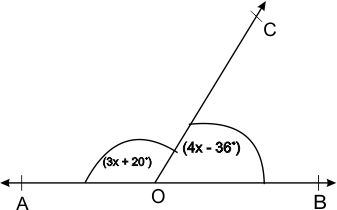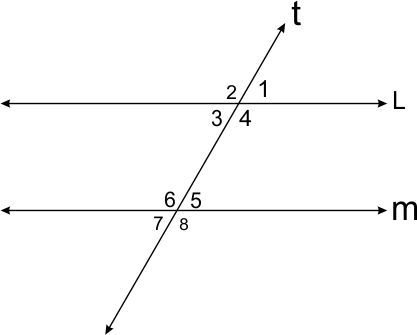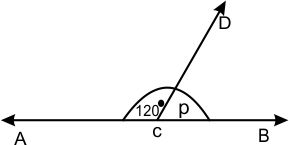6.9     TEST YOURSELF:

1. In the following figure, if AOB is a straight line then find the measure of        ∠AOC  and  ∠ BOC2. in the figure L II M and T it is a transversal , if    1 :    2 = 3: 7 then find the degree measures of all angles3. angles P and 100° form a linear pair. what is measure of P ?

4. in the adjoining figure what is the measure of P5. in the adjoining figure, AB II CD if    APQ = 54° and   PRD = 126°, then find X and Y